## Rational Function

A quotient of two polynomials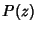and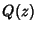,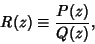is called a rational function. More generally, if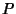and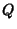are Polynomials in multiple variables, their quotient is a rational function.

See also Abel's Curve Theorem, Closed Form, Fundamental Theorem of Symmetric Functions, Quotient-Difference Algorithm, Rational Integer, Rational Number, Riemann Curve Theorem